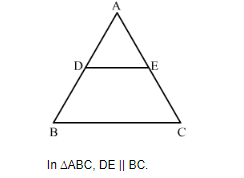# In ∆ABC, D and E are points on side AB and AC respectively such that DE || BC and AD : DB = 3 : 1. If EA = 3.3 cm, then AC =

Question:

In ∆ABC, D and E are points on side AB and AC respectively such that DE || BC and AD : DB = 3 : 1. If EA = 3.3 cm, then AC =

(a) 1.1 cm
(b) 4 cm
(c) 4.4 cm
(d) 5.5 cm

Solution:

Given: In ΔABC, D and E are points on the side AB and AC respectively such that DE || BC and AD : DB = 3 : 1. Also, EA = 3.3cm.

To find: ACUsing corollory of basic proportionality theorem, we have

$\frac{\mathrm{AD}}{\mathrm{AB}}=\frac{\mathrm{EA}}{\mathrm{AC}}$

$\frac{\mathrm{AD}}{\mathrm{AD}+\mathrm{BD}}=\frac{3.3}{\mathrm{AC}}$

$\frac{\mathrm{AD}}{\mathrm{AD}+\frac{1}{3} \mathrm{AD}}=\frac{3.3}{\mathrm{AC}}$

$\mathrm{EC}=4.4 \mathrm{~cm}$

Hence the correct answer is C